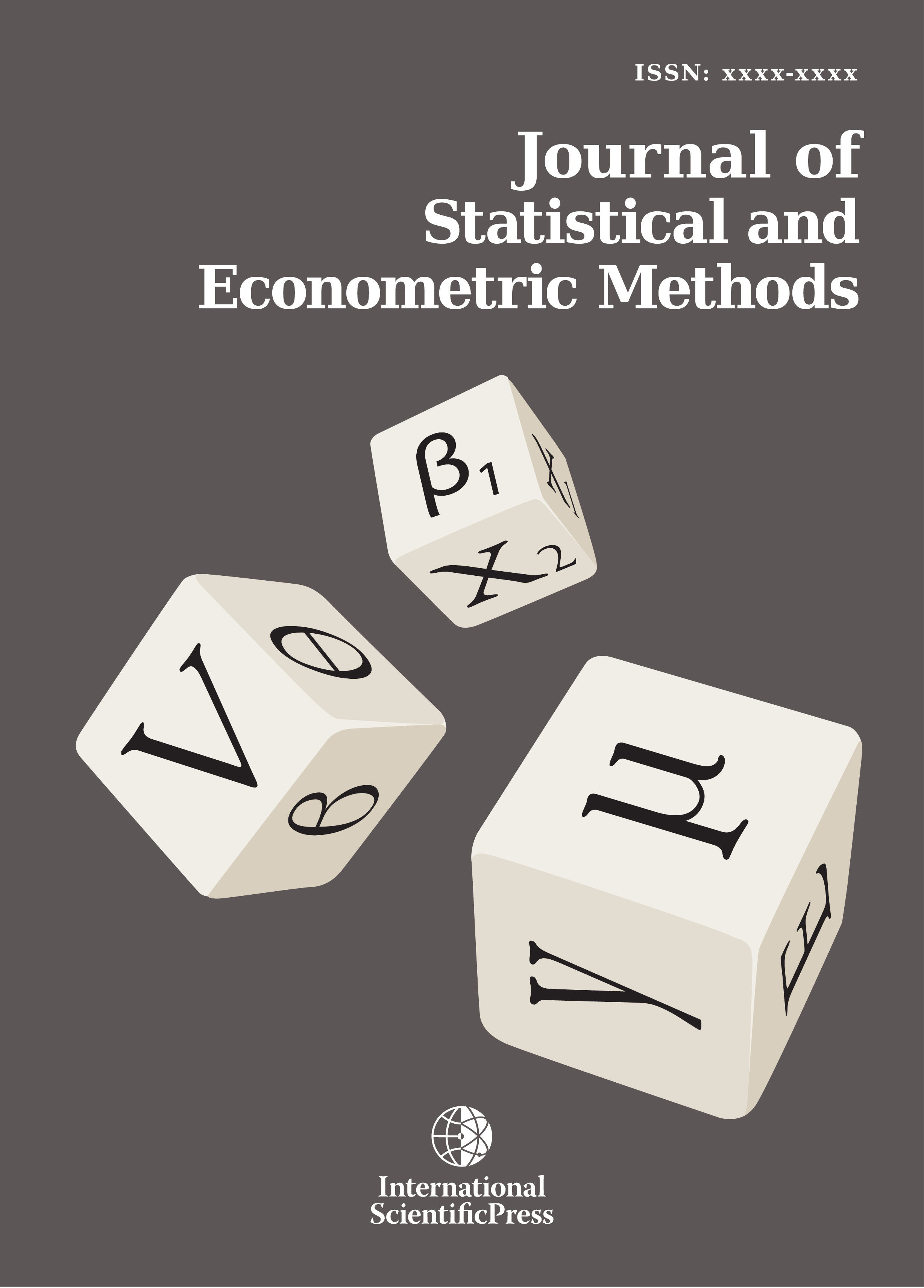# Journal of Statistical and Econometric Methods

#### Determination of lag threshold on the measure of collinearity

•[ Download ]
• Times downloaded: 1077
•                                                  Abstract

To date, the implementation of lag selection procedures within the context of unit root tests is largely based on application of standard information criteria which are well-known to have lag selection problem. In this paper, we propose a new lag selection procedure based on collinearity measures for an autoregression of differenced lagged values. In empirical analysis, the conventional coefficient of multiple determination denoted by Rc2 has been widely used in statistical literature for the computation of standard collinearity measures such as variance inflation factor (VIF) and tolerance index(ToL) but given the well-known difference between the underlying assumptions of standard multiple linear regression and a linear autoregression, the conventional coefficient of multiple determination which is used to compute VIF and ToL under the framework of standard multiple linear regression may not provide an appropriate collinearity measures under a linear autoregression setting. Hence, the realization of this obvious inadequacy motivated the introduction of our newly proposed statistic called the coefficient of multiple determination for autoregression of differenced lagged values of time series data. Unit root tests are implemented on autoregression made up of differenced lagged values which requires an appropriate choice of optimal truncation lag. However, in the current literature there is dearth of information on possibility of using collinearity statistics as criteria for lag truncation in unit root tests. Using the newly proposed coefficient of multiple determination for autoregression of differenced lagged series denoted by Rd2 , we generate a class of collinearity statistics called tolerance index and variance inflation factor for differenced lagged series denoted by ToLd and VIFd respectively. We used these collinearity statistics as criteria for determining lag threshold for unit root tests. We employed augmented Dickey-Fuller(ADF) and generalized Dickey Fuller (DF-GLS) unit root tests to illustrate empirically the application of these collinearity criteria for lag selection and we demonstrated that these criteria select lag-length that is parsimonious thereby avoiding the problem of over-specification of truncation lag-length that is commonly associated with classical lag selection techniques.ISSN: 2241-0376 (Online)
2241-0384 (Print)